1. Which of the following will reduce random errors in an experiment?
2. The best estimate for the time it takes light to cross the nucleus of the hydrogen atom is
3. Which is a unit of force?
4. Which of the following is a derived unit?
5. Which of the following is equivalent to the joule?
6. A boy jumps from a wall 3m high. What is an estimate of the change in momentum of the boy when he lands without rebounding?
7. The acceleration of free fall g is determined by the relationship

g=(4pi^2l)/t^2. The uncertainty in the value of l is 2% and the uncertainty in the value of t is 5%. What is the uncertainty in g?

8. The resistive force F acting on a sphere of radius r travelling with speed v through a liquid is given by the equation

F = 6pietarv

where ηis a constant. What are the SI units ofη?

9. The sides of a square are measured to be 5.0 ± 0.2 cm. Which of the following gives the area of the square and its uncertainty?
10. A small object is attached to a string and rotated in a circle of constant radius in a horizontal plane. The tension T in the string is measured for different speeds v. Which of the following plots should give a straight-line graph?
11. A stone falls from rest to the bottom of a water well of depth d. The time t taken to fall is 2.0 ±0.2 s. The depth of the well is calculated to be 20 m using

d=1/2at^2. The uncertainty in a is negligible.

What is the absolute uncertainty in d?

12. The length of each side of a sugar cube is measured as 10 mm with an uncertainty of ± 2 mm . Which of the following is the absolute uncertainty in the volume of the sugar cube?
13. Which of the following lists three vector quantities?
14. Which of the following expresses the watt in terms of fundamental units?
15. Which of the following is a fundamental unit?
16. The maximum acceleration

amax of an oscillator undergoing simple harmonic motion (SHM) has a percentage uncertainty of 12%. The amplitude x0 of the oscillation has a percentage uncertainty of 20%. ifk=sqrt(a_(max)/(x0) what is the percentage uncertainty in the constant k?

17. How many significant figures are there in the number 0.0450?
18. The radius of a sphere is measured with an uncertainty of 2%. What is the uncertainty in the volume of the sphere?
19. Which of the following is a scalar quantity?
20. A student measures the radius r of a sphere with an absolute uncertainty Δr. What is the fractional uncertainty in the volume of the sphere?
21. What is the unit of energy density?
22. A swimming pool contains

18×106 kg of pure water. The molar mass of water is 18gmol–1. What is the correct estimate of the number of water molecules in the swimming pool?

23. An object is positioned in a gravitational field. The measurement of gravitational force acting on the object has an uncertainty of 3 % and the uncertainty in the mass of the object is 9 %. What is the uncertainty in the gravitational field strength of the field?
24. A car moves north at a constant speed of 3m

s–1 for 20s and then east at a constant speed of 4m s–1 for 20s. What is the average speed of the car during this motion?

25. What is the correct SI unit for momentum?
26. The diagram below shows the forces acting on a block of weight W as it slides down a slope. The angle between the slope and the horizontal is θ, the normal reaction force on the block from the slope is N and friction is negligible.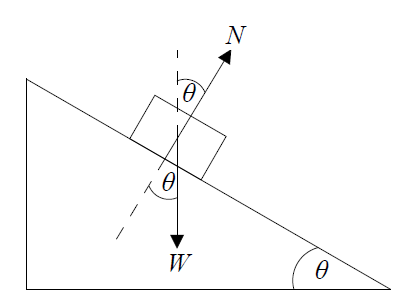Which of the following gives the resultant force on the block?

27. What is the best estimate for the diameter of a helium nucleus?
28. Which of the following lists two scalar quantities?
29. Which of the following is a unit of energy?
30. A body accelerates from rest with a uniform acceleration a for a time t. The uncertainty in a is 8% and the uncertainty in t is 4%. The uncertainty in the speed is
31. The force of air resistance F that acts on a car moving at speed v is given by

F=kv2 where k is a constant. What is the unit of k?

32. The masses and weights of different objects are independently measured. The graph is a plot of weight versus mass that includes error bars.These experimental results suggest that the

33. An object slides down an inclined plane that makes an angle θ with the horizontal. The weight of the object is W.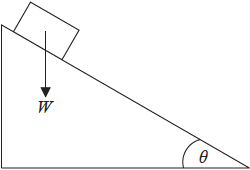Which of the following is the magnitude of the component of the weight parallel to the plane?

34. What is the order of magnitude of the mass, in kg, of an apple?
35. A volume is measured to be

52 mm3. This volume in m3 is

36. Which of the following is a fundamental SI unit?
37. The length of the side of a cube is 10.0 ±0.3cm. What is the uncertainty in the volume of the cube?
38. Which is a vector quantity?
39. Two pulses are travelling towards each other.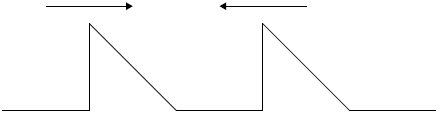What is a possible pulse shape when the pulses overlap?

40. Light of wavelength 400nm is incident on two slits separated by 1000µm. The interference pattern from the slits is observed from a satellite orbiting 0.4Mm above the Earth. The distance between interference maxima as detected at the satellite is
41. What is the unit of electrical energy in fundamental SI units?
42. An object falls for a time of 0.25 s. The acceleration of free fall is

9 .81 ms−2. The displacement is calculated. Which of the following gives the correct number of significant digits for the calculated value of the displacement of the object?

43. A velocity of 5 m

s−1 can be resolved along perpendicular directions XY and XZ.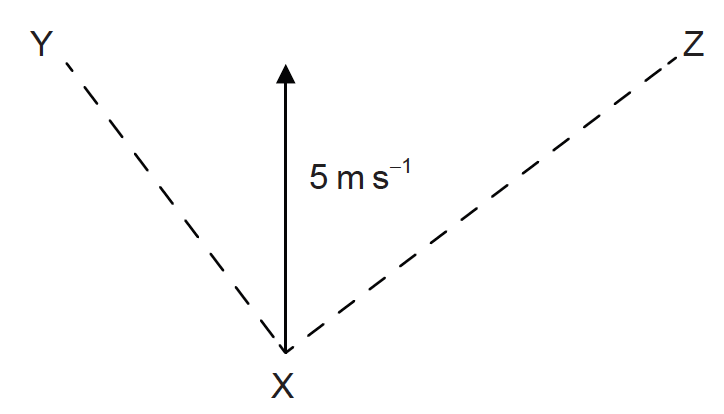The component of the velocity in the direction XY is of magnitude 4 m s−1. What is the magnitude of the component in the direction XZ?

44. The diagram shows an analogue meter with a mirror behind the pointer.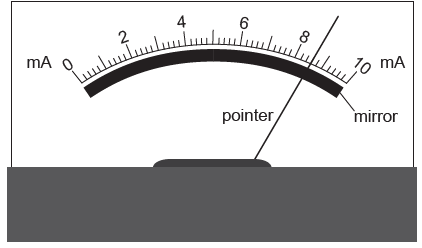What is the main purpose of the mirror?

45. The graph shows the relationship between two quantities p and q. The gradient of the graph is r and the intercept on the p axis is s.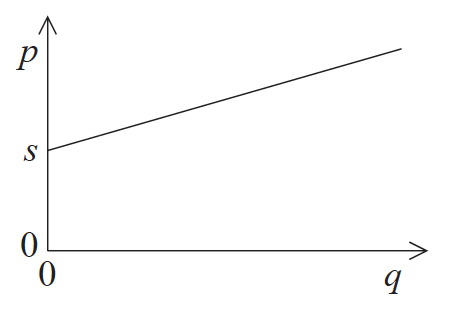Which of the following is the correct relationship between p and q?

46. Which of the following contains one fundamental and one derived unit?
47. The current I through a resistor is measured with a digital ammeter to be 0.10 A. The uncertainty in the calculated value of

I2 will be

48. A stone attached to a string is moving in a horizontal circle. The constant speed of the stone is v. The diagram below shows the stone in two different positions, X and Y.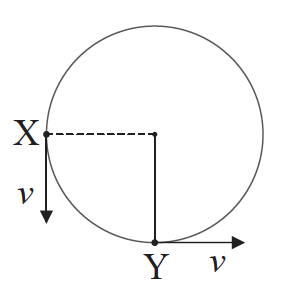49. The vector diagram shows two forces acting on a point object O. The forces are in the plane of the page.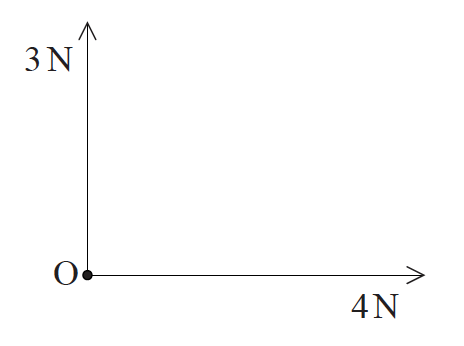Another 5 N force is applied to O in the plane of the page. Which of the following gives the direction of this force to ensure that O is in equilibrium?

50. Aiming for the centre of a target, an archer fires arrows which produces a pattern of hits as shown below.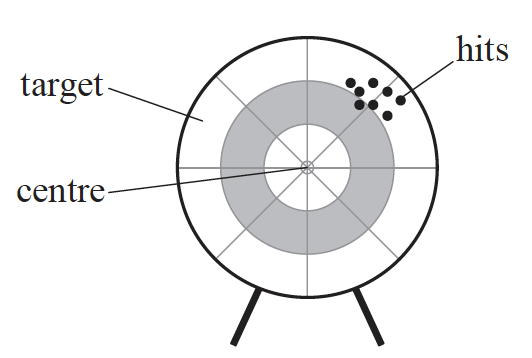The pattern suggests the presence of

51. The graph shows a set of experimental results to determine the density of oil. The results have systematic errors and random errors.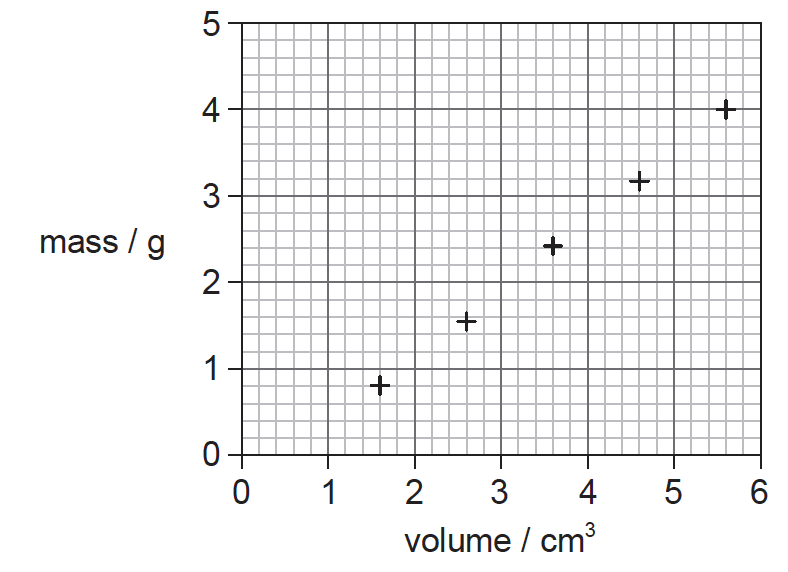Using the information on the graph, what can be said about the measurements used to find the density of oil?52. A sphere fits inside a cube.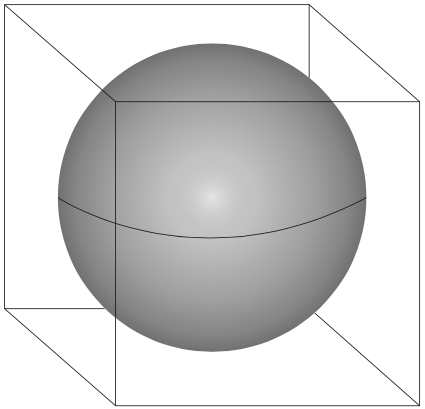The length of the cube and the diameter of the sphere are 10.0±0.2cm.

What is the ratio